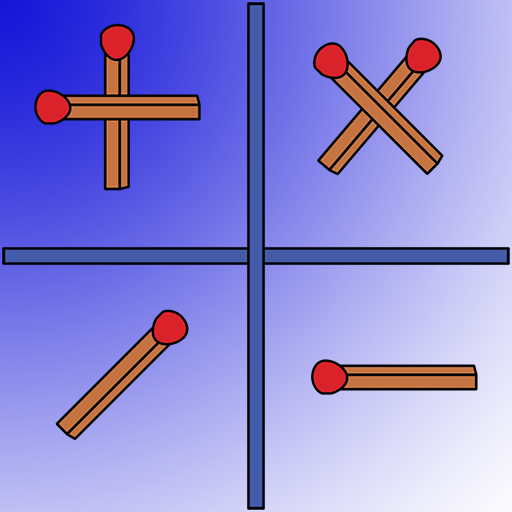# Voting Now

##### Creativity
×## Matches. Matchstick math game.

### by Andre Logic Puzzles

Free
Design
Usability
Creativity
Average
6 USERS VOTED
##### TAGS
###### Description

Matches Math Puzzle is a logic puzzle game with solving mathematical expressions by moving sticks. The game is divided into categories of varying difficulty. The game develops logical thinking and improves mathematical skills.
Math puzzles have three game modes:
1. Drag one stick to make the math equation correct.
2. Drag two matches.
3. Add the right amount of matches.
Also in Matches Math Puzzle - mathematical expressions are divided into categories:
• Simple actions (addition and subtraction)
• Multiplication table
• Double expressions
• All in one (all math operations)

For beginners, it will be easiest to start with categories in which you need to move just one matchstick.
More experienced users will be more interested in game modes with moving two matchsticks.
Adding the required number of matchsticks mode is a unique mode in which the user can find several different solutions to a mathematical problem.

Math matches is a good game for your brain.

View All